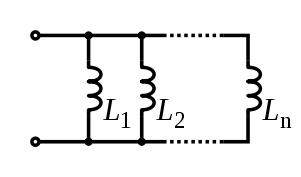## Friday, April 29, 2011

### Inductor & Transformer

1. INDUCTOR
An inductor is a coil of wire which may have a core of air, iron or ferrite (a brittle material made from iron). Its electrical property is called inductance and the unit for this is the henry, symbol H. 1H is very large so mH and µH are used, 1000µH = 1mH and 1000mH = 1H. Iron and ferrite cores increase the inductance. Inductors are mainly used in tuned circuits and to block high frequency AC signals (they are sometimes called chokes). They pass DC easily, but block AC signals, this is the opposite of capacitors.
An inductor may be connected either way round and no special precautions are required when soldering.

A. Picture
Inductor with air core :

Inductor with iron core:

Inductor with Ferrite core:

Variable inductor:

B. Symbol
Inductor with air core:

Inductor with iron or ferrite core:

Variable inductor:

C. Inductor Marking
- printed 102 = mean 1000 micro henry (µH) = 1 milihenry (mH)
- printed 105 = mean 1000000 microHenry = 1000miliHenry = mean 1 Henry
-color code :

D. Inductor Network
Inductors in a parallel configuration each have the same potential difference (voltage). To find their total equivalent inductance (Leq):The current through inductors in series stays the same, but the voltage across each inductor can be different. The sum of the potential differences (voltage) is equal to the total voltage. To find their total inductance:

These simple relationships hold true only when there is no mutual coupling of magnetic fields between individual inductors.

2. TRANSFORMER
A. Picture

Transformer Low voltage Singlephase:

Transformer High Voltage Multiphase:

B. Symbol

C. Basic Principles

If the secondary coil is attached to a load that allows current to flow, electrical power is transmitted from the primary circuit to the secondary circuit. Ideally, the transformer is perfectly efficient; all the incoming energy is transformed from the primary circuit to the magnetic field and into the secondary circuit. If this condition is met, the incoming electric power must equal the outgoing power:

giving the ideal transformer equation

P = Electric Power
Vp= Primary AC Voltage
Ip= Primary Current
Vs= Secondary AC Voltage
Is= Secundary current
Np= Turns of Primary
Ns= Turns of Secondary

D. Types of Transformer

In Generally Transformer can be classified :

1.Power Transformer
- Step Up Transformer
- Step Down Transformer

2. Instrument transformers
- Voltage Transformer
- Current Transformer
Used for metering and protection in high-voltage circuits.